Courses

Limits, Continuity and Differentiability Notes | Study Engineering Mathematics - GATE

GATE: Limits, Continuity and Differentiability Notes | Study Engineering Mathematics - GATE

The document Limits, Continuity and Differentiability Notes | Study Engineering Mathematics - GATE is a part of the GATE Course Engineering Mathematics.
All you need of GATE at this link: GATE

Limits

For a function f(x) the limit of the function at a point x = a is the value the function achieves at a point which is very close to x = a.
Formally,
Let f(x) be a function defined over some interval containing x = a, except that it may not be defined at that point.
We say that,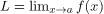if there is a number δ for every number ϵ such that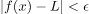whenever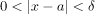The concept of limit is explained graphically in the following image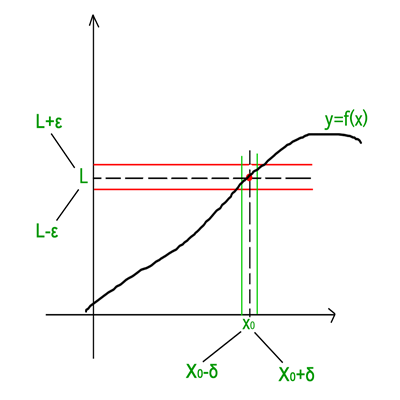As is clear from the above figure, the limit can be approached from either sides of the number line i.e. the limit can be defined in terms of a number less that a or in terms of a number greater than a.
Using this criteria there are two types of limits:

1. Left Hand Limit: If the limit is defined in terms of a number which is less than a then the limit is said to be the left hand limit. It is denoted as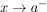which is equivalent to x = a - h where h > 0 and h → 0.
2. Right Hand Limit: If the limit is defined in terms of a number which is greater than a then the limit is said to be the right hand limit. It is denoted as x → a+ which is equivalent to x = a + h where h > 0 and h → 0.

Existence of Limit: The limit of a function f(x) at x = a exists only when its left hand limit and right hand limit exist and are equal and have a finite value i.e.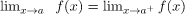Some Common Limits: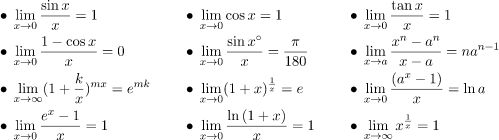L’Hospital Rule

If the given limit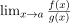is of the form 0/0 or ∞/∞ i.e. both f(x) and g(x) are 0 or both f(x) and g(x) are ∞ then the limit can be solved by L’Hospital Rule.
If the limit is of the form described above, then the L’Hospital Rule says that –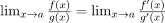where f'(x) and g'(x) obtained by differentiating f(x) and g(x).
If after differentitating, the form still exists, then the rule can be applied continuously until the form is changed.

Example 1: Evaluate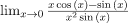Solution: The limit is of the form 0/0, Using L’Hospital Rule and differentiating numerator and denominator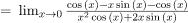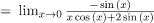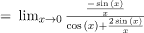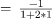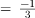Example 2: Evaluate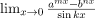Solution: On multiplying and dividing by kx and re-writing the limit we get –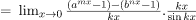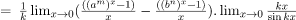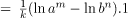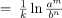Continuity

A function is said to be continuous over a range if it’s graph is a single unbroken curve.
Formally,
A real valued function f(x) is said to be continuous at a point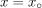in the domain if –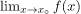f(x) exists and is equal to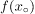.
If a function f(x) is continuous atthen-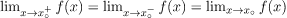Functions that are not continuous are said to be discontinuous

Example: For what value of λ is the function defined by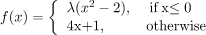continuous at x = 0?

Solution: For the function to be continuous the left hand limit, right hand limit and the value of the function at that point must be equal.

Value of function at x=0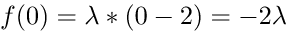Right hand limit-

=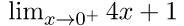= 1

RHL equals value of function at 0-

-2λ = 1

λ = -1 / 2

Differentiability

The derivative of a real valued function f(x) wrt x is the function f'(x) and is defined as –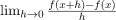A function is said to be differentiable if the derivative of the function exists at all points of its domain. For checking the differentiability of a function at point x = c,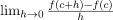must exist.
If a function is differentiable at a point, then it is also continuous at that point.

Note: If a function is continuous at a point does not imply that the function is also differentiable at that point. For example, f(x) = |x| is continuous at x = 0 but it is not differentiable at that point.

The document Limits, Continuity and Differentiability Notes | Study Engineering Mathematics - GATE is a part of the GATE Course Engineering Mathematics.
All you need of GATE at this link: GATEUse Code STAYHOME200 and get INR 200 additional OFF Use Coupon CodeEngineering Mathematics

54 docs|21 tests

Track your progress, build streaks, highlight & save important lessons and more!

,

,

,

,

,

,

,

,

,

,

,

,

,

,

,

,

,

,

,

,

,

,

,

,

;